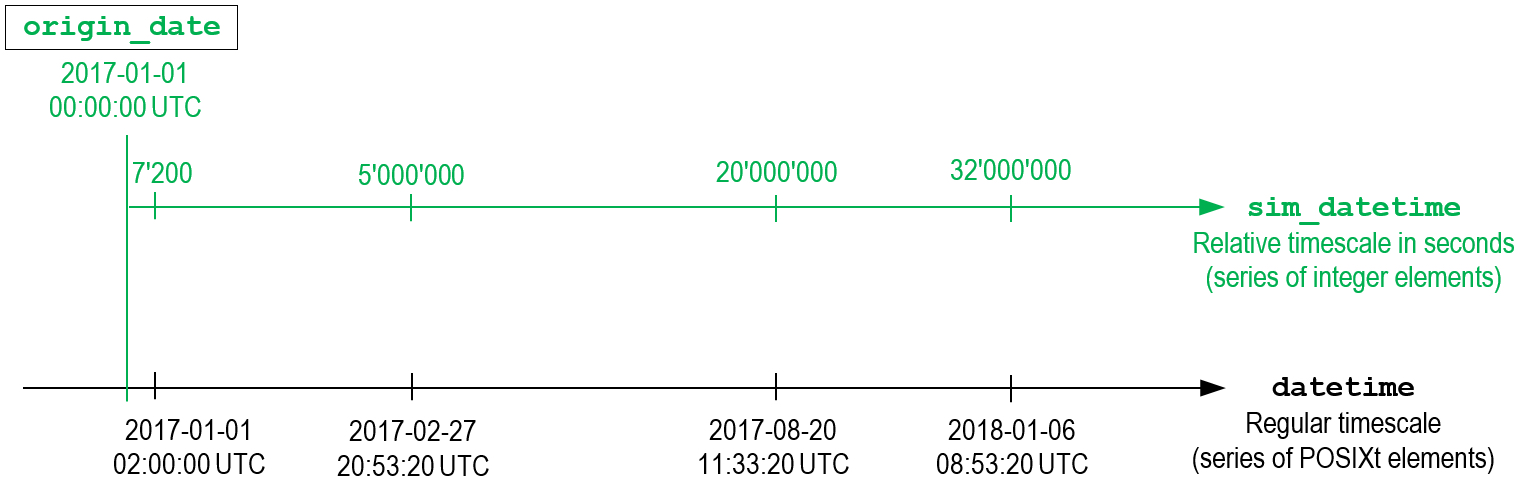# simtimer

#### 2019-01-22

simtimer is a little R package designed to simplify (and speeding up) calculating time intervals in discrete event simulations.

Discrete event simulations is a simulation paradigm that is based on the evaluation of events taking place in a time-specific order. Therefore a discrete event simulation calculates many time intervals. simtimer handles dates and times as integers. This makes working with time intervals as easy (and as fast) as subtracting integers.

simtimer uses an origin_date and calculates all dates and times referring to that origin_date.

The applications of simtimer are discrete event simulations representing a timerange of several minutes to years. For such problems, the time dimension might preferably be represented as a relative timescale (integer) than a series of date_time objects (POSIXt). simtimer is a basic tool to transform date_times to a relative timescale in seconds (sim_datetime) and vice versa. Additionally simtimer allows to extract elements of a sim_datetime such as time, weekday and date.## Basic transformation

For the transformation into the relative timescale (date_time -> sim_datetime) use the corresponding function as.sim_datetime(). This function expects an origin_date of choice to be predefined. This origin_date should be earlier in time than the following date_time-elements.

library(simtimer)
origin_date <- as.POSIXct("2017-01-01 00:00:00", tz = "UTC")
my_datetime <- c(as.POSIXct("2017-01-01 02:00:00", tz = "UTC"),
as.POSIXct("2017-02-27 20:53:20", tz = "UTC"),
as.POSIXct("2017-08-20 11:33:20", tz = "UTC"),
as.POSIXct("2018-01-06 08:53:20", tz = "UTC"))
my_sim_datetime <- as.sim_datetime(my_datetime, origin_date)
my_sim_datetime
##      7200  5000000 20000000 32000000
class(my_sim_datetime)
##  "integer"

All remaining functions of simtimer take a sim_datetime as argument.

For the opposite transformation (sim_datetime -> date_time) use the corresponding function as.datetime() with the same origin_date.

as.datetime(my_sim_datetime, origin_date)
##  "2017-01-01 02:00:00 UTC" "2017-02-27 20:53:20 UTC"
##  "2017-08-20 11:33:20 UTC" "2018-01-06 08:53:20 UTC"

## Extracting the time (in seconds)

You can extract the time (in seconds) of a sim_datetime element by using the function sim_time(). It returns how many seconds have past since the last “beginning of a day” depending on the time-part of your origin_date (“00:00:00” is recommended).

sim_time(my_sim_datetime)
##   7200 75200 41600 32000

## Extracting the weekday

You can extract the weekday of a sim_datetime elemet by using the function sim_wday(). It returns the weekday of your sim_datetime.

sim_wday(my_sim_datetime, origin_date)
##  "7" "1" "7" "6"

## Extracting the date

In order to count the days that have passed since the beginning (your origin_date) use sim_date(). To be more precise - the function returns the number of 24*60*60-intervals that have passed since your origin_date.

sim_date(my_sim_datetime)
##    0  57 231 370